# GMAT Math : DSQ: Calculating the midpoint of a line segment

## Example Questions

### Example Question #671 : Geometry

A line segment has one of its endpoints at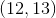. In which quadrant, or on what axis, is its other endpoint?

Statement 1: The midpoint of the segment is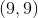.

Statement 2: The length of the segment is 10.

EITHER statement ALONE is sufficient to answer the question.

BOTH statements TOGETHER are sufficient to answer the question, but NEITHER statement ALONE is sufficient to answer the question.

Statement 2 ALONE is sufficient to answer the question, but Statement 1 ALONE is NOT sufficient to answer the question.

Statement 1 ALONE is sufficient to answer the question, but Statement 2 ALONE is NOT sufficient to answer the question.

BOTH statements TOGETHER are insufficient to answer the question.

EITHER statement ALONE is sufficient to answer the question.

Explanation:

Statement 1 give us the means to find the other endpoint using the midpoint formula: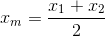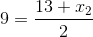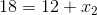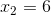Similarly,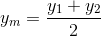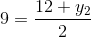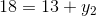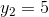This makes the endpoint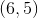, which is in Quadrant I.

Statement 2 is also sufficient., which is in Quadrant 1, is 12 units away from the nearest axis; since the length of the segment is 10, the entire segment must be in Quadrant I.

### Example Question #672 : Geometry

In what quadrant or axis is the midpoint of the line segment with endpoints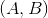and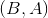located?

Statement 1: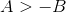Statement 2:is in Quadrant IV.

Statement 1 ALONE is sufficient to answer the question, but Statement 2 ALONE is NOT sufficient to answer the question.

Statement 2 ALONE is sufficient to answer the question, but Statement 1 ALONE is NOT sufficient to answer the question.

BOTH statements TOGETHER are insufficient to answer the question.

EITHER statement ALONE is sufficient to answer the question.

BOTH statements TOGETHER are sufficient to answer the question, but NEITHER statement ALONE is sufficient to answer the question.

Statement 1 ALONE is sufficient to answer the question, but Statement 2 ALONE is NOT sufficient to answer the question.

Explanation:

The midpoint of the segment with endpointsandis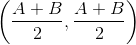.

If, then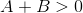and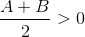, so the midpoint, having both of its coordinates positive, is in Quadrant I.

Ifis in Quadrant IV, then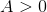and. But the quadrant of the midpoint varies according toand:

Example 1: If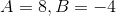, the midpoint is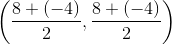, or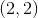, putting it in Quadrant I.

Example 2: If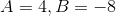, the midpoint is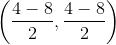, or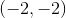, putting it in Quadrant III.

Therefore, the first statement, but not the second, tells us all we need to know.

### Example Question #673 : Geometry

Consider segment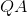. What are the coordinates of the midpoint of?

I) Point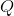has coordinates of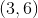.

II) Pointhas coordinates of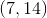.

Neither statement is sufficient to solve the question. More information is needed.

Statement 1 is sufficient to solve the question, but statement 2 is not sufficient to solve the question.

Each statement alone is enough to solve the question.

Both statements taken together are sufficient to solve the question.

Statement 2 is sufficient to solve the question, but statement 1 is not sufficient to solve the question.

Both statements taken together are sufficient to solve the question.

Explanation:

We are asked to find the midpoint of a line segment and given endpoints in our clues.

Midpoint formula is found by taking the average of the x and y values of two points.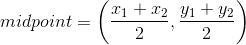We need both endpoints to solve this problem, so both statements are needed.

### Example Question #681 : Geometry

Find the midpoint of segment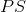given that pointis at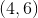.

I) Thecoordinate of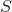is twice that of, and thecoordinate ofis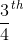that of.

II)is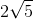units long.

Statement II is sufficient to answer the question, but Statement I is not sufficient to answer the question.

Either statement alone is sufficient to answer the question.

Both statements together are needed to answer the question.

Statement I is sufficient to answer the question, but Statement II is not sufficient to answer the question.

Statement I is sufficient to answer the question, but Statement II is not sufficient to answer the question.

Explanation:

To find the midpoint, we need to know both endpoints.

I) Gives us the means to find out other endpoint.

II) Gives us the length of PS, but we are not given any hint as to its orientation.Thus, we cannot find the other endpoint and we cannot find the midpoint.

Thus, Statement I alone is sufficient to answer the question.

### Example Question #682 : Geometry

Find the midpoint of segment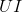.

I) Endpoint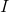has coordinates of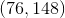.

II) Endpoint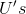coordinate is half of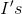, andcoordinate is one sixteenth ofcoordinate.

Either statement is sufficient to answer the question.

Statement I is sufficient to answer the question, but statement II is not sufficient to answer the question.

Both statements are needed to answer the question.

Statement II is sufficient to answer the question, but statement I is not sufficient to answer the question.

Both statements are needed to answer the question.

Explanation:

To find the midpoint of a segment we need both endpoints

I) Gives us one endpoint.

II) Gives us clues to find the other endpoint.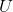has coordinates of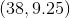Use midpoint formula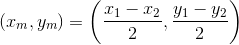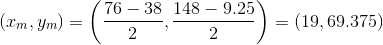Tired of practice problems?

Try live online GMAT prep today.